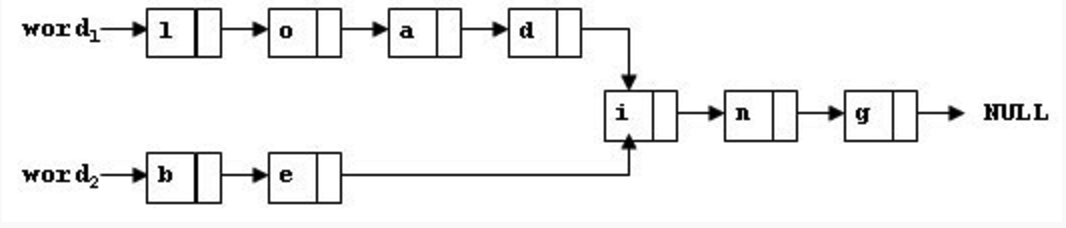# 1032. Sharing (25)-PAT甲级真题

To store English words, one method is to use linked lists and store a word letter by letter. To save some space, we may let the words share the same sublist if they share the same suffix. For example, “loading” and “being” are stored as showed in Figure 1.Figure 1
You are supposed to find the starting position of the common suffix (e.g. the position of “i” in Figure 1).

Input Specification:

Each input file contains one test case. For each case, the first line contains two addresses of nodes and a positive N (<= 105), where the two addresses are the addresses of the first nodes of the two words, and N is the total number of nodes. The address of a node is a 5-digit positive integer, and NULL is represented by -1.

Then N lines follow, each describes a node in the format:

where Address is the position of the node, Data is the letter contained by this node which is an English letter chosen from {a-z, A-Z}, and Next is the position of the next node.

Output Specification:

For each case, simply output the 5-digit starting position of the common suffix. If the two words have no common suffix, output “-1” instead.

Sample Input 1:
11111 22222 9
67890 i 00002
00010 a 12345
00003 g -1
12345 D 67890
00002 n 00003
22222 B 23456
11111 L 00001
23456 e 67890
00001 o 00010
Sample Output 1:
67890
Sample Input 2:
00001 00002 4
00001 a 10001
10001 s -1
00002 a 10002
10002 t -1
Sample Output 2:
-1